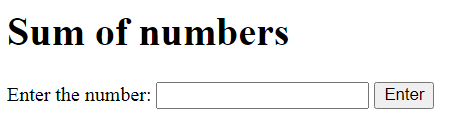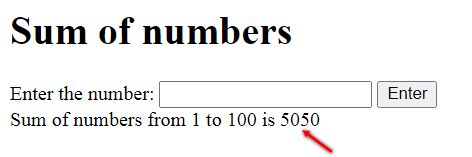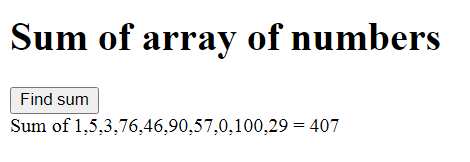# How to find the sum of numbers using a for loop in JavaScript?

In this tutorial, let’s learn how to find the sum of numbers using a for loop in JavaScript. It’s a very simple solution, so let’s quickly look at it.

## Sum of numbers within a range using a for loop

To start with, we’re going to create our HTML page. We’re going to have a h1 tag that displays the title.

I’d like to get user input for the range. Then, we can calculate the sum of numbers from 1 to that range. We can also do the same with an array of numbers. Let’s look at that next.

Now, for this example, let’s create an input box of id ‘range’.

Then, we need a button of id ‘button’. When the user presses on this button, the sum of the given numbers should be displayed in the div element we create right after. This div element is going to have an id of ‘output’.

We’re going to manipulate this div element to display the sum of the numbers right after the user presses the button.

```    <h1>Sum of numbers</h1>
Enter the number: <input id='range' type="text" />
<button id='button'>Enter</button>
<div id="output"></div>
```

Now, let’s get the range, button and div element by their ids and place them in constants. We’re placing these elements in constants, and not variables because we won’t be reassigning these values again in this program.

Let’s also create variables ‘r’ and ‘sum’ and assign them values of 0 respectively. ‘r’ is going to contain the user specified range, and ‘sum’ is going to contain the sum of numbers.

```const range = document.getElementById('range');
const button = document.getElementById('button');
const output = document.getElementById('output');

let r = 0, sum = 0;
```

Now, let’s add a new click event listener to the ‘button’ element. Let’s create an anonymous arrow function that specifies what happens when the button is clicked on.

`button.addEventListener('click',() => {`

To start with, we need to extract the value in the ‘range’ element. Since we created a text input, let’s use the parseInt method to convert the text into a number.

`    r = parseInt(range.value,10);`

Then, let’s create a for loop that iterates through 1 to r. For every iteration, let’s add the current value of ‘i’, which starts from 1, to the ‘sum’ variable.

We’re going to use the ‘+=’ to add the new value of ‘i’ to the old value of ‘sum’. Now you understand why we declared ‘sum’ with a value of 0. We did that so we have a number, albeit one with no value, that can be added to the first value of ‘i’.

```    for(let i=1; i<=r; i++) {
sum += i;
}
```

Once the for loop is done, we can print it to our page by changing the innerText of the ‘output’ variable.

Let’s also reset the value of our ‘range’ element into an empty string and reassign the value 0 to our ‘sum’ variable.

The entire function is as follows:

```button.addEventListener('click',() => {
r = parseInt(range.value,10);
for(let i=1; i<=r; i++) {
sum += i;
}
output.innerText = `Sum of numbers from 1 to \${r} is \${sum}`;
range.value = '';
sum = 0;
});
```

That’s it! Now, let’s run the program and look at the output.

Before the user enters the range and presses the button, this is what they see:Let’s enter 100 and press ‘Enter’, and we should get:## Find the sum of array of numbers

Now, let’s try to do the same with an array of numbers. We’re going to have to remove our input box for this example, since we don’t need to get user input now. Let us retain the button and the div element that’ll display the output.

```    <h1>Sum of array of numbers</h1>
<button id='button'>Find sum</button>
<div id="output"></div>
```

Next, let’s work on our script file. Let’s create an array of random numbers, like the one below.

```let arr = [1,5,3,76,46,90,57,0,100,29];

Then, let’s retrieve the 2 elements, and create a variable ‘sum’ that’s assigned to 0 to start with.

const button = document.getElementById('button');
const output = document.getElementById('output');

let sum = 0;
```

Finally, let’s create the click event listener that’ll find the sum of the array. Unlike the earlier example, since we’re handling an array of numbers in here, we need to iterate through the length of that array.

So, let’s create a for loop that iterates from 0 to right before the length of the array, which can be found with the array.length property.

For every iteration, let’s add the current value of ‘sum’ to the element in the ‘ith’ position of the array. At the end of the loop, we’ll have the sum of all the numbers in the array, which is exactly what we want.

Let’s update the innerText of the ‘output’ element and end the program.

```button.addEventListener('click',() => {
for(let i=0; i<arr.length; i++) {
sum += arr[i];
}
output.innerText = `Sum of \${arr} = \${sum}`;
});
```

When you run the above lines of code, you’ll see:There you go! This is how you can find the sum of numbers using for loop, be it within a range or within an array.